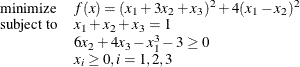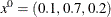### A Simple Problem

Consider the following simple example of a nonlinear optimization problem:The problem consists of a quadratic objective function, a linear equality constraint, and a nonlinear inequality constraint. The goal is to find a local minimum, starting from the point. You can use the following call to PROC OPTMODEL to find a local minimum:

proc optmodel;
var x{1..3} >= 0;
minimize f = (x + 3*x + x)**2 + 4*(x - x)**2;

con constr1: sum{i in 1..3}x[i] = 1;
con constr2: 6*x + 4*x - x**3 - 3 >= 0;

/* starting point */
x = 0.1;
x = 0.7;
x = 0.2;

solve with NLP;
print x;
quit;


Because no options have been specified, the default solver (INTERIORPOINT) is used to solve the problem. The SAS output displays a detailed summary of the problem along with the status of the solver at termination, the total number of iterations required, and the value of the objective function at the local minimum. The summaries and the optimal solution are shown in Figure 8.1.

Figure 8.1: Problem Summary, Solution Summary, and the Optimal Solution

The OPTMODEL Procedure

Problem Summary
Objective Sense Minimization
Objective Function f
Objective Type Quadratic

Number of Variables 3
Bounded Above 0
Bounded Below 3
Bounded Below and Above 0
Free 0
Fixed 0

Number of Constraints 2
Linear LE (<=) 0
Linear EQ (=) 1
Linear GE (>=) 0
Linear Range 0
Nonlinear LE (<=) 0
Nonlinear EQ (=) 0
Nonlinear GE (>=) 1
Nonlinear Range 0

Performance Information
Execution Mode Single-Machine
Number of Threads 4

Solution Summary
Solver NLP
Algorithm Interior Point
Objective Function f
Solution Status Optimal
Objective Value 1.000017384

Optimality Error 5E-7
Infeasibility 2.5088265E-8

Iterations 5
Presolve Time 0.00
Solution Time 0.03

 x
1 0.0003034484
2 0.0000042439
3 0.9996923328

The SAS log shown in Figure 8.2 displays a brief summary of the problem being solved, followed by the iterations that are generated by the solver.

Figure 8.2: Progress of the Algorithm as Shown in the Log

 NOTE: Problem generation will use 4 threads. NOTE: The problem has 3 variables (0 free, 0 fixed). NOTE: The problem has 1 linear constraints (0 LE, 1 EQ, 0 GE, 0 range). NOTE: The problem has 3 linear constraint coefficients. NOTE: The problem has 1 nonlinear constraints (0 LE, 0 EQ, 1 GE, 0 range). NOTE: The OPTMODEL presolver removed 0 variables, 0 linear constraints, and 0 nonlinear constraints. NOTE: Using analytic derivatives for objective. NOTE: Using analytic derivatives for nonlinear constraints. NOTE: The NLP solver is called. NOTE: The Interior Point algorithm is used. Objective                          Optimality Iter             Value     Infeasibility             Error 0        7.20000000                 0        6.40213404 1        1.22115550        0.00042385        0.00500000 2        1.00188693        0.00003290        0.00480263 3        1.00275609        0.00002123        0.00005000 4        1.00001702   0.0000000252254        0.00187172 5        1.00001738   0.0000000250883   0.0000005000000 NOTE: Optimal. NOTE: Objective = 1.000017384.